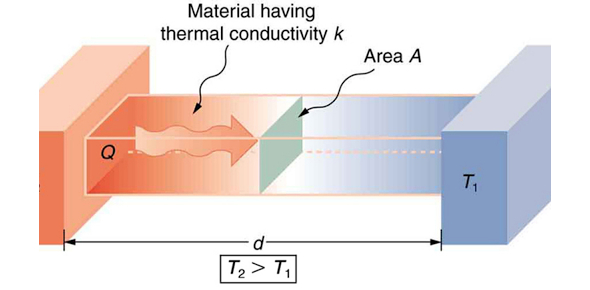# Thermal Physics Quiz Exam!

8 Questions | Total Attempts: 127Settings.

• 1.
Which of the following graph represent the motion when Abby throw an apple onto the floor at 0s. (under free fall assumption)
• A.

I

• B.

II

• C.

III

• D.

IV

• 2.
Abby is travel from Pà Q àRàS. What is the average speed traveled by Abby?
• A.

5 m/s

• B.

1.380 m/s

• C.

5000 m/s

• D.

1380 m/s

• 3.
Given point P is the pivot of the level. Determine its direction of rotation.
• A.

Clockwise

• B.

Anticlockwise

• C.

Remain equilibrium

• 4.
Determine its direction of rotation.
• A.

Clockwise

• B.

Anticlockwise

• C.

No rotation occur

• D.

Option 4

• 5.
A lit match is placed above a thermometer. State the type of thermal processes where the heat from the match reaches the thermometer.
• A.

Conduction

• B.

Convection

• C.

• 6.
Which process involves convection?
• A.

• B.

Energy from the Sun warming a road surface

• C.

Hot air rising to the top of a cool room

• D.

Thermal energy transfer through a copper bar

• 7.
The air in a room is heated by a heater. The diagram shows the circulation of the air in the room. Which statement about the air that is heated is correct?
• A.

The air contracts and becomes less dense.

• B.

The air contracts and becomes more dense.

• C.

The air expands and becomes less dense.

• D.

The air expands and becomes more dense.

• 8.
Given the level is under an equilibrium state. Find the value of x.
• A.

1.27 m

• B.

0.6 m

• C.

2.67 m

• D.

5.93 m

Related TopicsBack to top